# Grouping by Age Range

I have a formula field, "Age Range" with this formula:

IF(VALUE(Age__c)>18,"19+",
IF(VALUE(Age__c)>=15,"15-18",
IF(VALUE(Age__c)>=10,"10-14",
IF(VALUE(Age__c)>=6,"6-9",
IF(VALUE(Age__c)>=3,"3-5",
IF(VALUE(Age__c)<=2,"None", "None"))))))

This age range shows up great in a report, but I want to include it in a dashboard.   I have an aggregate model and want to group by this age range field, but age range doesn't show up in my groupings - it will only let me aggregate this field.  Any thoughts on why?

• edited September 1, 2016
This is a limitation that Salesforce imposes on its Aggregate queries.  Very frustrating.

The only way we have gotten around this is by creating a text field that uses workflow rules to get its values from the formula field.   Its a real hack (And probably violates some clause of a salesforce contract)  but its how we've gotten this to work.
• Doh!  That is frustrating....and obviously challenging my lil brain.  :-(

I created a new text field called Age_Range_Text.  I created my workflow rule: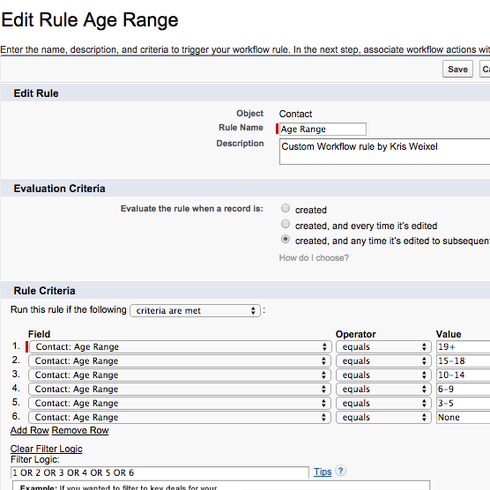And set the action to update my text field: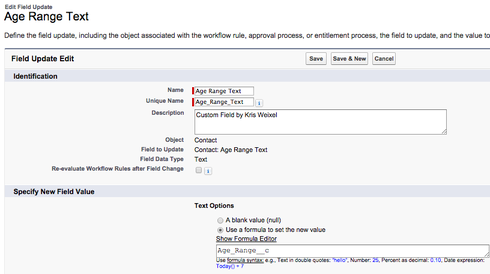But i get notta....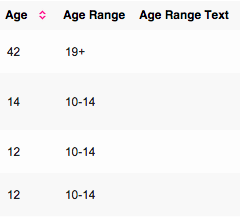Do you see what i'm doing wrong?

Thanks for all your help!!!!  I appreciate it SOOOOO much!
Kris

• edited September 1, 2016
Here is a success community discussion about moving a field using a workflow rule.  Not really a Skuid item, but here you go.  https://success.salesforce.com/answers?id=90630000000h3XMAAY

• Change the workflow to 'created and every time is edited' (second option), setting setting the third option (subsequently bla bla bla) its only triggered when the conditions were evaluated to false and after the edit are evaluated to true.

your condition is always true (always is in a age range) so is never triggered
• OK....i got this to work now.  Thank you!!!
• Any thoughts though on how to sort a text field w/ numbers though?  My model is using the Age_Range_Text__c for the field to sort by (advanced tab of the model), but it still shows up in random order.  I haven't found how to change this for a text field like this...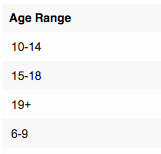• Could you add another field for each of these that would be an index for each age range, so 6-9 would have an index of 1, 10-14 an index of 2 and so on, You could then sort by that field in the advanced properties? You could also then show the values in a template field and still use the index field to do the ordering so that it can be re-ordered in the table.
• Hi Oliver,

Thank you for your response....  I think I'm confused.  Right now, to try and make this work, I have:
1. Birthdate  - Date field
2. Age - formula field to calculate age based on birthdate
3. Age range - formula field to put that age into a defined range
4. Age range - text field to put the age range formula field into a text field so i can use it in a skuid chart
I have these 4 fields so far.  Are you saying that instead of step 4, I need to add 4 more fields - 1 text field for each range w/ each defined index and then add each individual field to the chart?

All of this seems a lil wonky just to get a range.  I haven't played with template fields much - would that be easier???

Thanks!
Kris

• edited September 1, 2016
You don't need to add 4 new fields.  You've got enough already!!

1.  6-9
2. 10-14
3. 15-18
4. 19+

What Oliver is suggesting is one more field that gives an index based on age.  Much like your other formula field,  except that instead of the text you have,  your output values are 1, 2, 3, etc.  What I fear however is that you will need to group this field as well,  and therefore will need to add a text field to go along with your formula field.

Complicated.

• Thanks, Rob.

OK, so i looked in salesforce at both the text and formula fields - I was looking to find out to add it to my formula, but the help files i found says i must have a where clause to index a field, but that doesn't seem to apply....?  I don't see where in the formula field to tell it to start w/ an index...

Cornfused....

• edited September 1, 2016
I think the word Index is sending you down a rabbit trail.   Your formula code needs to be somthing like:

If (AgeRange = "6-9", 1, If (AgeRange = "10-14", 2, If (AgeRange = "15-18", 3, 4)))
• Ahhh...yes, i was going down a rabbit trail.  Your formula makes sense to me....I'm close to getting this, but just 1 more question:

So, my age range field, Age_Range__c, has this calculation:

IF(VALUE(Age__c)>18,"19+",
IF(VALUE(Age__c)>=15,"15-18",
IF(VALUE(Age__c)>=10,"10-14",
IF(VALUE(Age__c)>=6,"6-9",
IF(VALUE(Age__c)>=3,"3-5",
IF(VALUE(Age__c)<=2,"None", "None"))))))

I have a workflow rule that takes the value from Age_Range__c  <> "" and updates field age range text, Age_Range_Text__c.

I tried to put your formula in the rule criteria for my workflow, but that doesn't work.  Should there be another formula field, like age range index, and use the field for my workflow?  I understand your formula, but think am still a lil unclear how / where to fit it into my what nots...  sorry for all my confusion!

Thanks!
Kris

• edited September 1, 2016
The criteria should not include the formula from your age range field.  The criteria should be somthing that basically brings in all records.

• hmmm.... i went through these steps again and am following what's outlined in this link.  my criteria is the same as what he has - 'Age_Range'  <> "" and I see my text field being populated correctly with the age ranges.

my question though is on your response below on the indexing and which field this formula should be included in?

You said:
Your formula code needs to be somthing like:

If (AgeRange = "6-9", 1, If (AgeRange = "10-14", 2, If (AgeRange = "15-18", 3, 4)))

Where do i put this?
•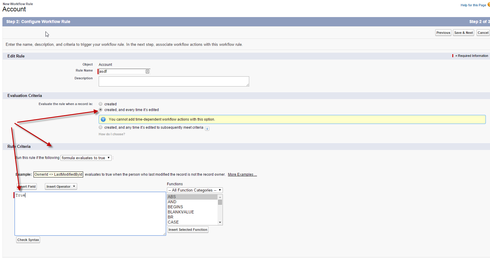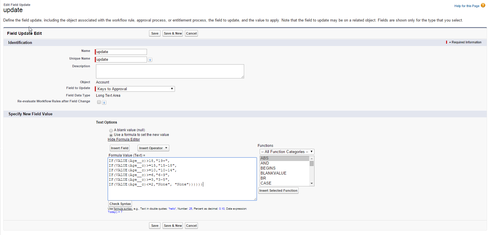• Thanks, Pat.  This part works - the field is being correctly updated w/ the range.

What's not working is the order of age ranges that appear in my skuid table.  The age ranges are random vs. the order i want.

To fix this random order in the skuid table, Rob posted a formula like this to use -
If (AgeRange = "6-9", 1, If (AgeRange = "10-14", 2, If (AgeRange = "15-18", 3, 4)))

I can do this, but where does this formula go?

1.  birthdate - date field

2.  age - formula field to calculate the age based on birthdate

IF(TODAY() >=Date(YEAR(TODAY()),
MONTH(Birthdate), DAY(Birthdate)),
TEXT(YEAR(TODAY()) - YEAR(Birthdate)),
TEXT(YEAR(TODAY()) - YEAR(Birthdate) - 1))

3.  age range - text field that uses the workflow exactly like you have above to put ages into a defined range for my skuid table

IF(VALUE(Age__c)>18,"19+",
IF(VALUE(Age__c)>=15,"15-18",
IF(VALUE(Age__c)>=10,"10-14",
IF(VALUE(Age__c)>=6,"6-9",
IF(VALUE(Age__c)>=3,"3-5",
IF(VALUE(Age__c)<=2,"None", "None"))))))

I'm confused because this formula to index these values - If (AgeRange = "6-9", 1, If (AgeRange = "10-14", 2, If (AgeRange = "15-18", 3, 4))) - has a formula return type of number.  This doesn't show up for me to group by in my skuid table because my table grouping wants a data return type of text....

I want to understand what do with this formula to sort the line items in my table -
If (AgeRange = "6-9", 1, If (AgeRange = "10-14", 2, If (AgeRange = "15-18", 3, 4)))

I realize that even though I'm trying to make this work in a skuid table, my answer is in salesforce.....so any help is appreciated!

• Yeah. You might have come to an end of what you can do for this requirement by declarative means. You can't use formulas as they are not allowed in aggregate models. You can use Workflows to work around this, but with one limitation. You can't use "Chained" workflow rule formulas as the values provided to a workflow rule will be based on the values existing in the record and not the values currently being calculated by another workflow rule. So, you can correctly calculate the Age Range, but not the subsequent sort index value for each Age Range.

One thing you can do is combine the Age Range label and desired sorting index number.

From:
IF(VALUE(Age__c)>18,"19+",
IF(VALUE(Age__c)>=15,"15-18",
IF(VALUE(Age__c)>=10,"10-14",
IF(VALUE(Age__c)>=6,"6-9",
IF(VALUE(Age__c)>=3,"3-5",
IF(VALUE(Age__c)<=2,"None", "None"))))))

To:
IF(VALUE(Age__c)>18,"5: 19+",
IF(VALUE(Age__c)>=15,"4: 15-18",
IF(VALUE(Age__c)>=10,"3: 10-14",
IF(VALUE(Age__c)>=6,"2: 6-9",
IF(VALUE(Age__c)>=3,"1: 3-5",
IF(VALUE(Age__c)<=2,"None", "0: None"))))))

It's not pretty but it would work.
• Thanks Pat.  Because demographics are really important for our funders and donors (we're a non-profit), I've been trying to create stuff in Skuid so i don't have to always manually do it in excel.  Unfortunately, this isn't pretty to be able to send a funder, so unless y'all know of any other tricks, we will just have to do this in excel.

Kris
• Kris,

It sounds like you want your table ordered by age range? I'm not sure if ORDER BY plays well with Aggregate models, but it's worth a shot if you haven't tried it.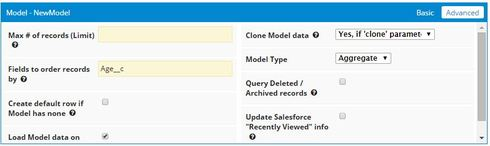• edited September 1, 2016
Thanks Matt.  The problem with the Order By and Aggregates is that the Ordering field has to be included in the Grouping.  Kris doesn't want a Grouping row for every age.

I'm not giving up on this though.  I think we can produce a solution for Kris.
• Ok, I just read through this whole thread. Can someone explain why adding AgeRange (the text field) to the ORDER BY clause doesn't produce the desired result?

Also, could Kris add a second field update to the workflow rule which would update an 'index' field?
• Thanks Matt & Rob.  I do have the AgeRange (text field) in the order by clause....

Matt - I'm thinking about what you're saying about the 2nd field update and think i have an idea of what you're saying and will try it....will let you know how it goes.

Thank you again soooo much!  I can't tell you how much I appreciate y'all and the support from this community!

Kris